Question

Please solve a,b,c,d,e,f 1) A water fountain is to be installed at a remote location by attaching a cast iron pipe directly to a water main through which water is flowing. The entrance to the pipe...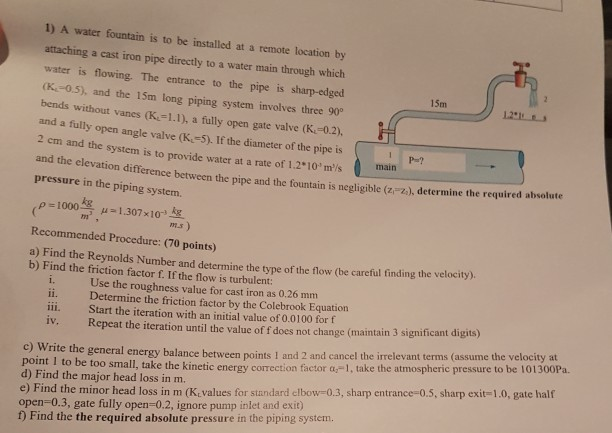1) A water fountain is to be installed at a remote location by attaching a cast iron pipe directly to a water main through which water is flowing. The entrance to the pipe is sharp-edged 15m (K-0.5), and the 15m long piping system involves three 90 bends without vanes (K1.1), a fully open gate valve (K.-0.2), and a fully open angle valve (K-5). If the diameter of the pipe is 2 em and the system is to provide water at a rate of 1.2 10 m''s and the elevation difference between the pipe and the fountain is negligible (z"z), determine the req P-? main pressure in the piping system. kg Recommended Procedure: (70 points) Find the Reynolds Number and determine the tyne of the flow (be careful finding the velocity). b) Find the friction factor f. If the flow is turbulent Use the roughness value for cast iron as 0.26 mm ii. Determine the friction factor by the Colebrook Equation ii. Start the iteration with an initial value of 0.0100 for f iv. Repeat the iteration until the value of f does not change (maintain 3 significant digits) e general energy balance between points I and 2 and cancel the irrelevant terms (assume the velocity at a,-1, take the atmospheric pressure to be 101300Pa. c) Write th point 1 to be too small, take the kinetic energy correction factor d) Find the major head loss in m. e) Find the minor head loss in m (K values for standard elbow 0.3, sharp entra open-0.3, gate fully open-0.2, ignore pump inlet and exit) f) Find the the required absolute pressure in the piping system. ce-0.5, sharp exit-1.0, gate half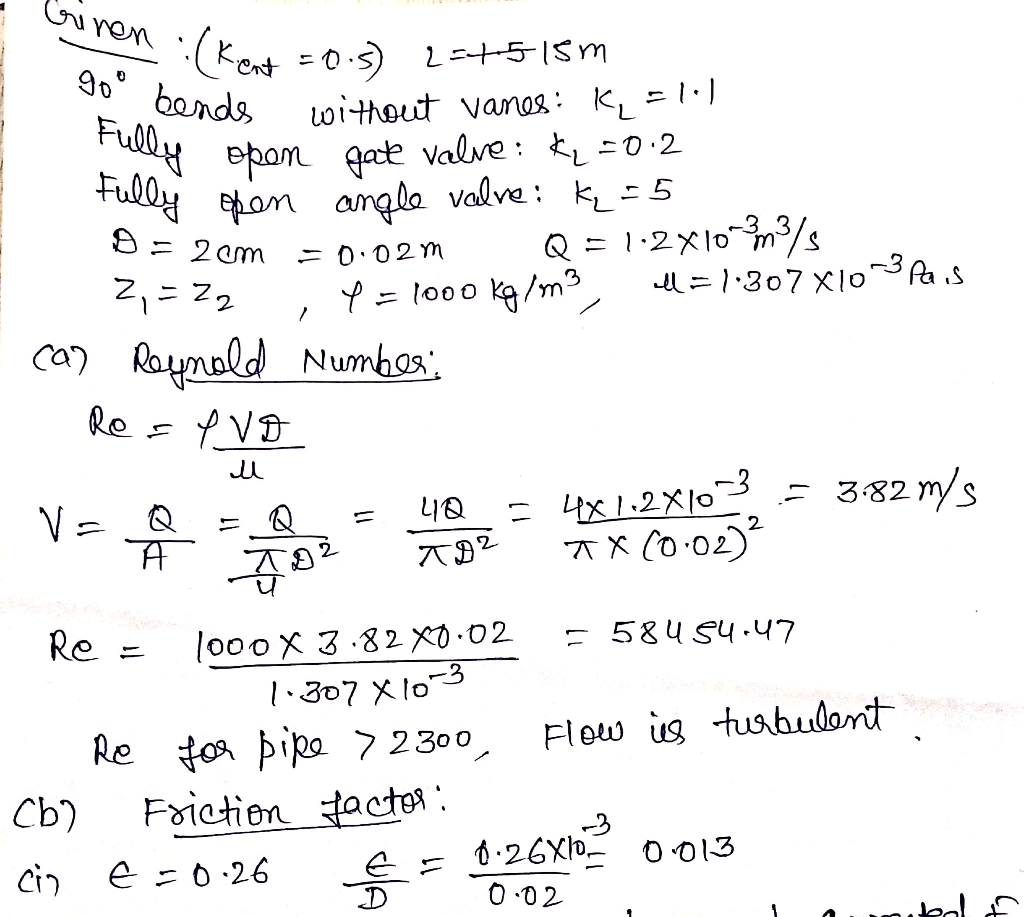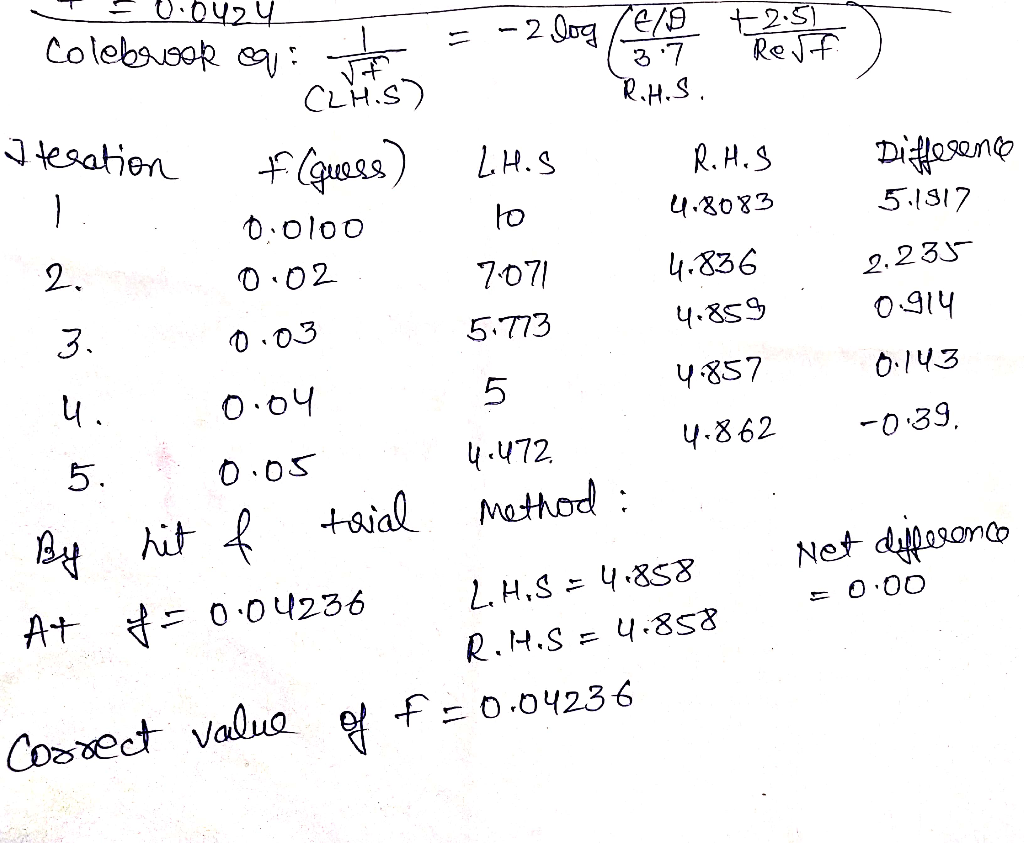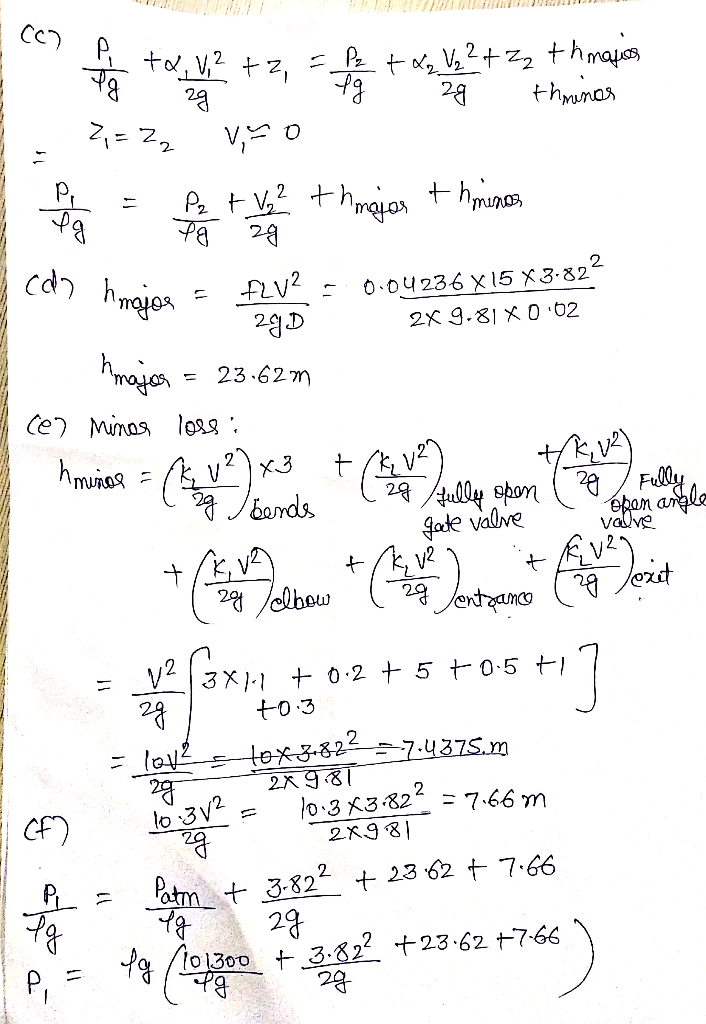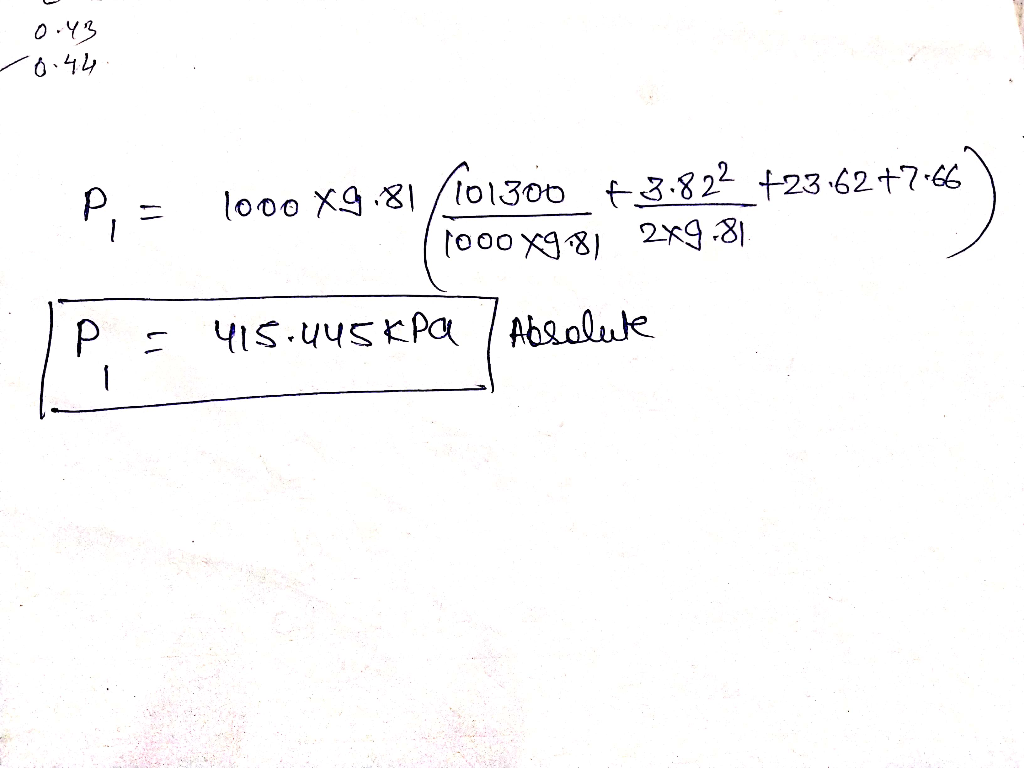Earn Coins

Coins can be redeemed for fabulous gifts.

Similar Homework Help Questions
• Two water reservoirs A and B are connected to each other through a 35m-long, 2-cm-diameter cast...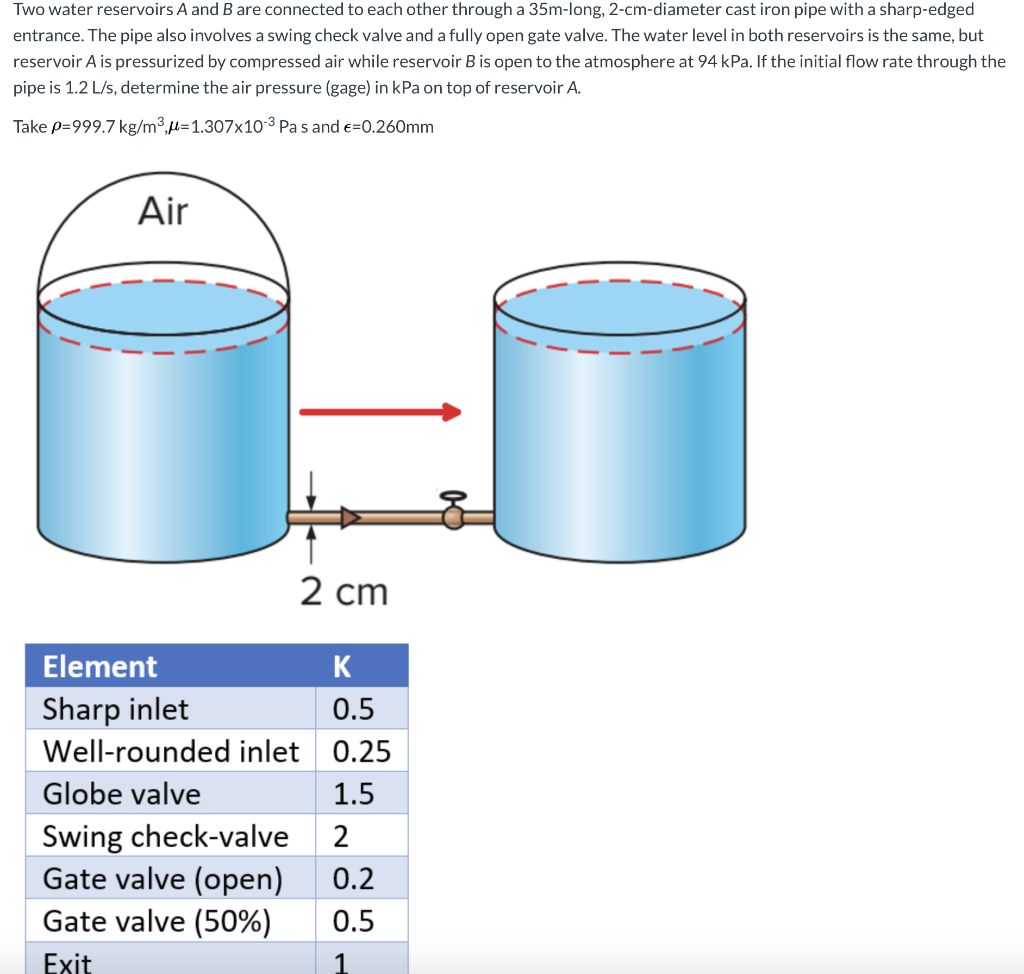Two water reservoirs A and B are connected to each other through a 35m-long, 2-cm-diameter cast iron pipe with a sharp-edged entrance. The pipe also involves a swing check valve and a fully open gate valve. The water level in both reservoirs is the same, but reservoir A is pressurized by compressed air while reservoir B is open to the atmosphere at 94 kPa. If the initial flow rate through the pipe is 1.2 L/s, determine the air pressure (gage)...

• 3) The system in Figure below consists of 1200 m of 5 cm cast-iron pipe, two...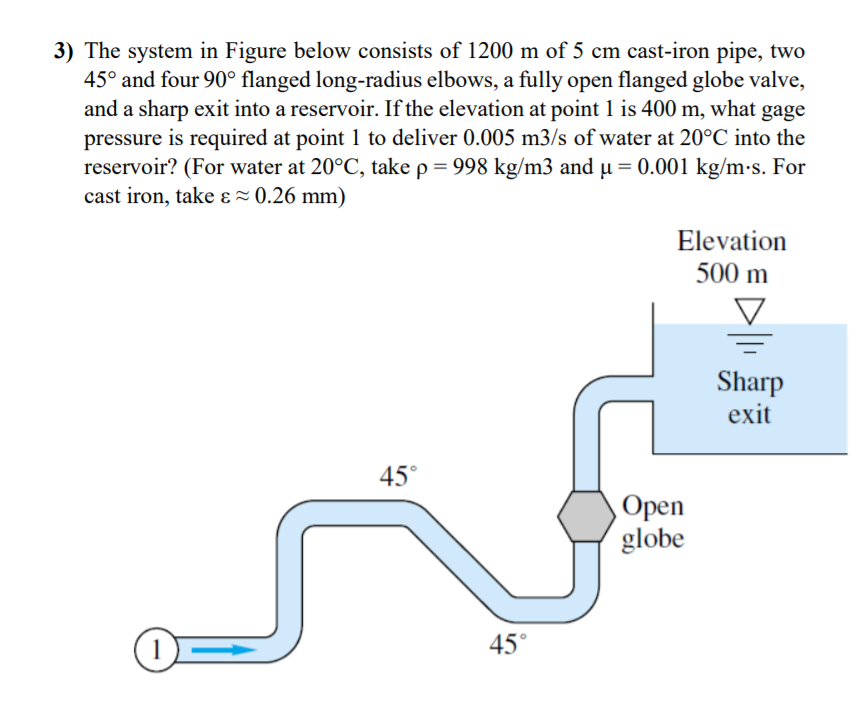3) The system in Figure below consists of 1200 m of 5 cm cast-iron pipe, two 45° and four 90° flanged long-radius elbows, a fully open flanged globe valve, and a sharp exit into a reservoir. If the elevation at point 1 is 400 m, what gage pressure is required at point 1 to deliver 0.005 m3/s of water at 20°C into the reservoir? (For water at 20°C, take p-998 kg/m3 and u-0.001 kg/m-s. For cast iron, take ε 0.26...

• The total length of the piping system made of cast iron providing hot water to a...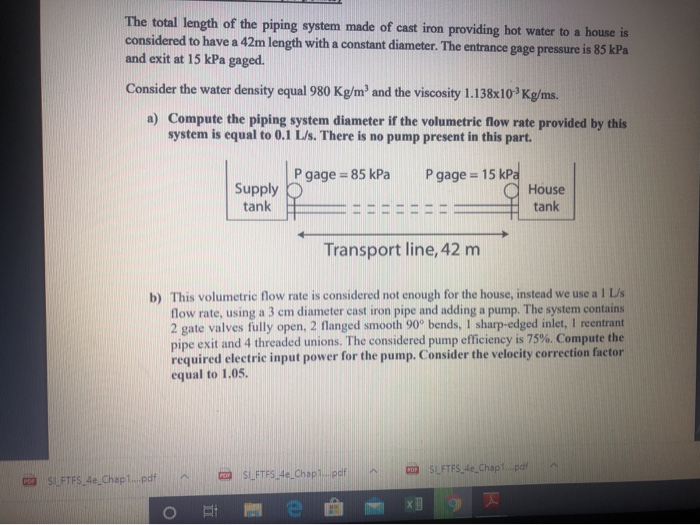The total length of the piping system made of cast iron providing hot water to a house is considered to have a 42m length with a constant diameter. The entrance gage pressure is 85 kPa and exit at 15 kPa gaged. Consider the water density equal 980 Kg/m' and the viscosity 1.138x10 Kg/ms. a) Compute the piping system diameter if the volumetric flow rate provided by this system is equal to 0.1 L/s. There is no pump present in this...

• Problem 5: The system in the Figure consists of 1200 m of 5 cm cast-iron pipe, two 45o and four 90 flanged long-radius elbows, a fully open flanged globe valve, and a sharp exit into a reservoir. If...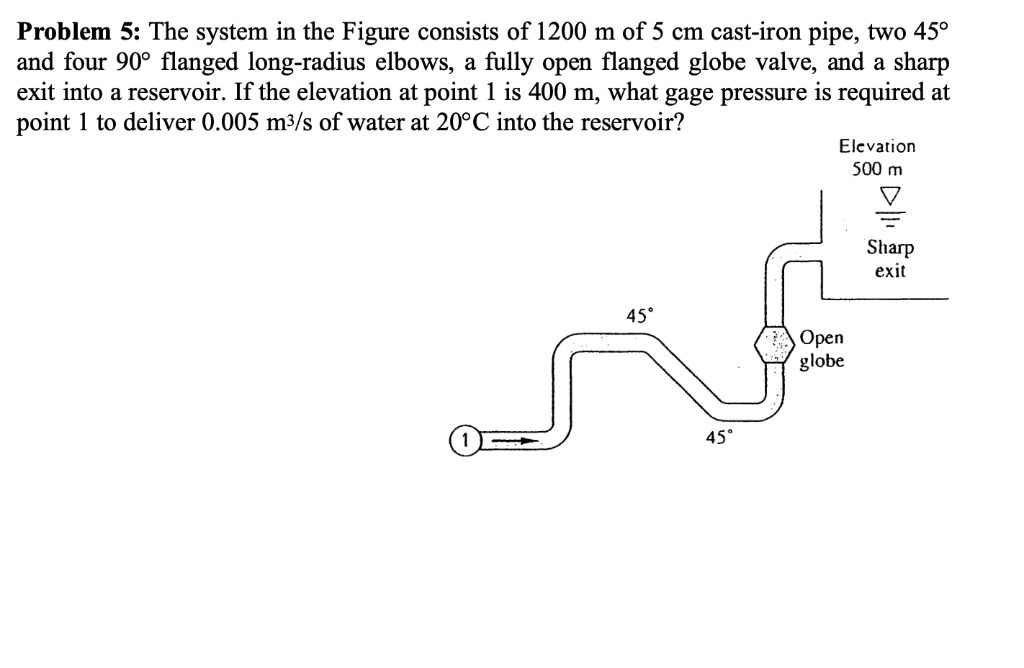Problem 5: The system in the Figure consists of 1200 m of 5 cm cast-iron pipe, two 45o and four 90 flanged long-radius elbows, a fully open flanged globe valve, and a sharp exit into a reservoir. If the elevation at point 1 is 400 m, what gage pressure is required at point 1 to deliver 0.005 m3/s of water at 20°C into the reservoir? Elevation 500 m Sharp exit 45° Open globe 45 Problem 5: The system in the...

• A 1-mile long 12-inch diameter asphalt dipped cast iron pipe (e = 0.00085 ft) conveys 60°F water at 3 cfs. Determine (a) friction factor f using the Moody Diagram, (b) f using the Colebrook equation,...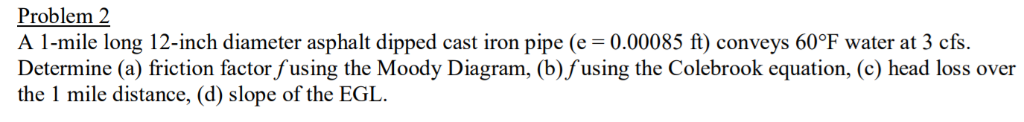A 1-mile long 12-inch diameter asphalt dipped cast iron pipe (e = 0.00085 ft) conveys 60°F water at 3 cfs. Determine (a) friction factor f using the Moody Diagram, (b) f using the Colebrook equation, (c) head loss over the 1 mile distance, (d) slope of the EGL. Problem 2 A 1-mile long 12-inch diameter asphalt dipped cast iron pipe (e = 0.00085 ft) conveys 60℉ water at 3 cfs Determine (a) friction factor fusing the Moody Diagram, (b) fusing...

• The system shown in the diagram consists of 1200m of cast-iron pipe with an internal diameter...

The system shown in the diagram consists of 1200m of cast-iron pipe with an internal diameter of 5cm (roughness of 0.00015 ft), two 45o and four 90o long radius elbows, a fully open bevel seat globe valve, and a sharp exit into a reservoir. If the elevation difference between the inlet and level in the reservoir is 10m, what gauge pressure is required at point 1 to deliver 0.005m^3/s of water at 20oC into the reservoir (use density of 998.2...

• Two water reservoirs A and B are connected to each other through a 35m-long, 2-cm-diameter cast...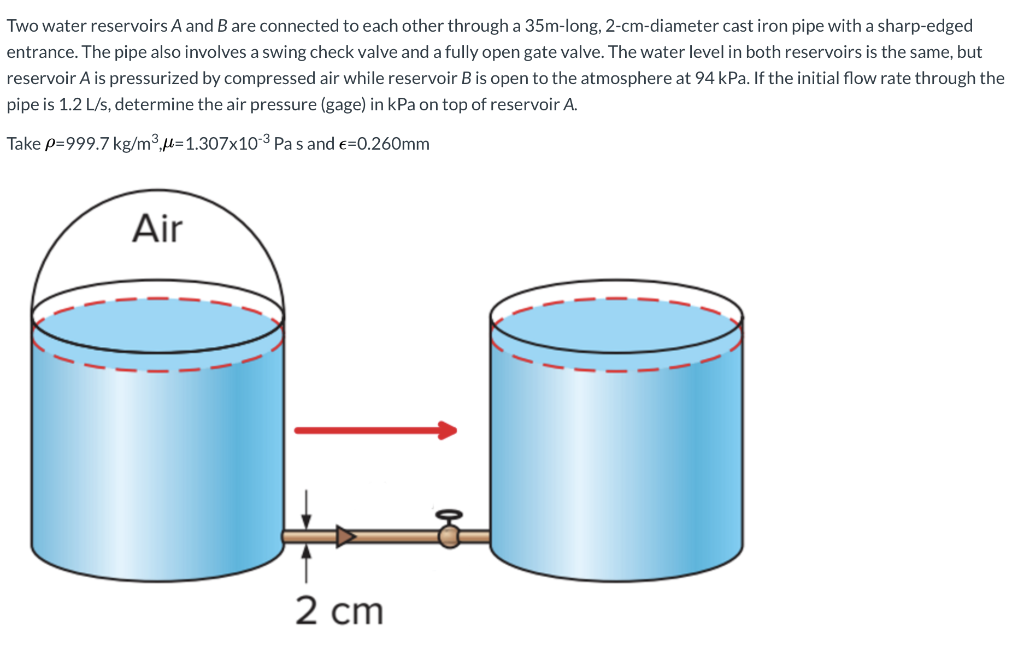Two water reservoirs A and B are connected to each other through a 35m-long, 2-cm-diameter cast iron pipe with a sharp-edged entrance. The pipe also involves a swing check valve and a fully open gate valve. The water level in both reservoirs is the same, but reservoir A is pressurized by compressed air while reservoir B is open to the atmosphere at 94 kPa. If the initial flow rate through the pipe is 1.2 L/s, determine the air pressure (gage)...

• A pump transports water from Tank 1 to Tank 2 through a constant-diameter piping system as...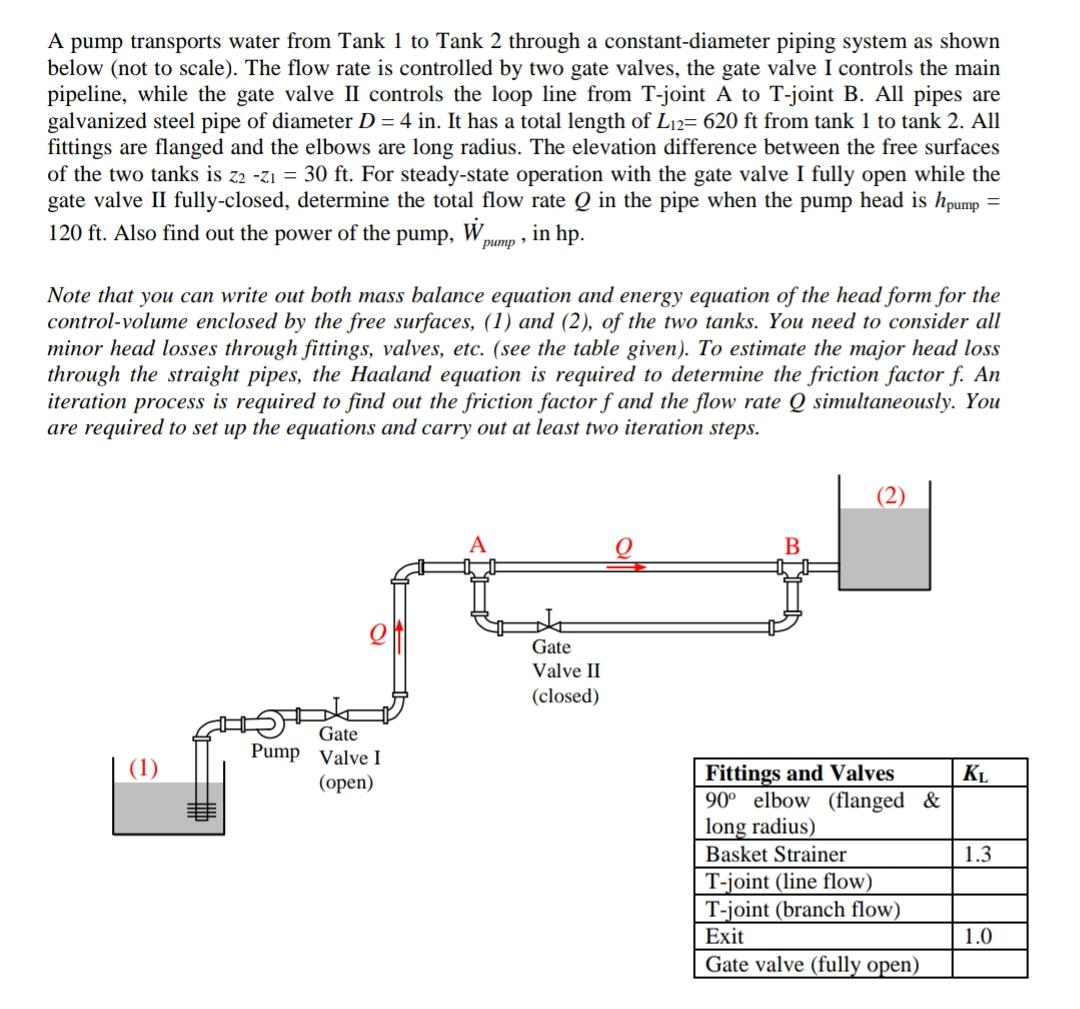A pump transports water from Tank 1 to Tank 2 through a constant-diameter piping system as shown below (not to scale). The flow rate is controlled by two gate valves, the gate valve I controls the main pipeline, while the gate valve II controls the loop line from T-joint A to T-joint B. All pipes are galvanized steel pipe of diameter D = 4 in. It has a total length of Li2= 620 ft from tank 1 to tank 2....

• this is all of the information given Problem 3. A piping system consists of 1200 m...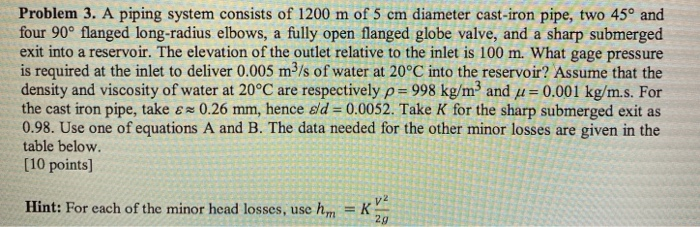this is all of the information given Problem 3. A piping system consists of 1200 m of 5 cm diameter cast-iron pipe, two 45° and four 90° flanged long-radius elbows, a fully open flanged globe valve, and a sharp submerged exit into a reservoir. The elevation of the outlet relative to the inlet is 100 m. What gage pressure is required at the inlet to deliver 0.005 m2/s of water at 20°C into the reservoir? Assume that the density and...

• Water flows from the upper tank to the lower tank through a cast iron pipe of total length L-1100 ft through one half-closed gate valve, four regular 90° flanged elbows, and entrance/exit as shown. F...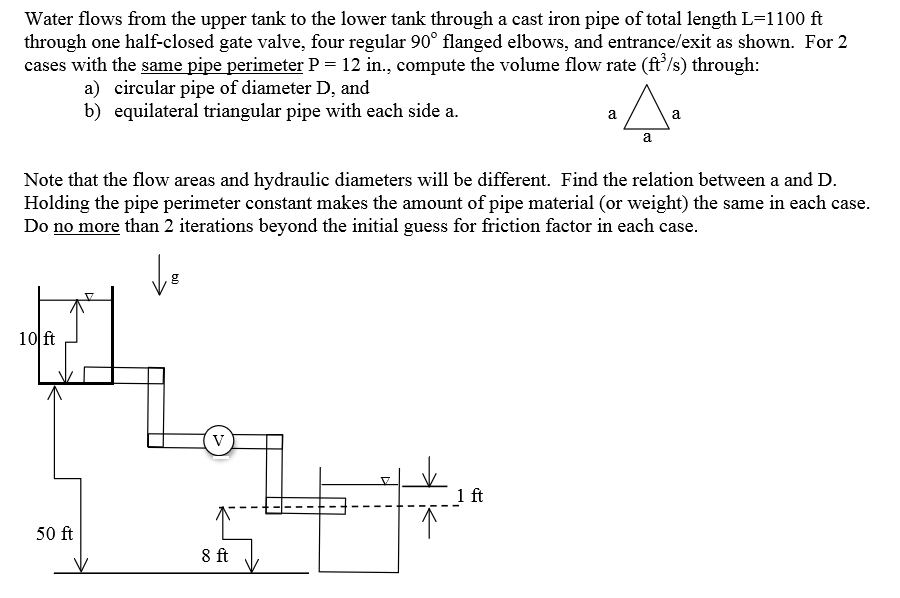Water flows from the upper tank to the lower tank through a cast iron pipe of total length L-1100 ft through one half-closed gate valve, four regular 90° flanged elbows, and entrance/exit as shown. For 2 cases with the same pipe perimeter P 12 in., compute the volume flow rate (ft /s) through: circular pipe of diameter D, and equilateral triangular pipe with each side a. a) b) Note that the flow areas and hydraulic diameters will be different. Find...